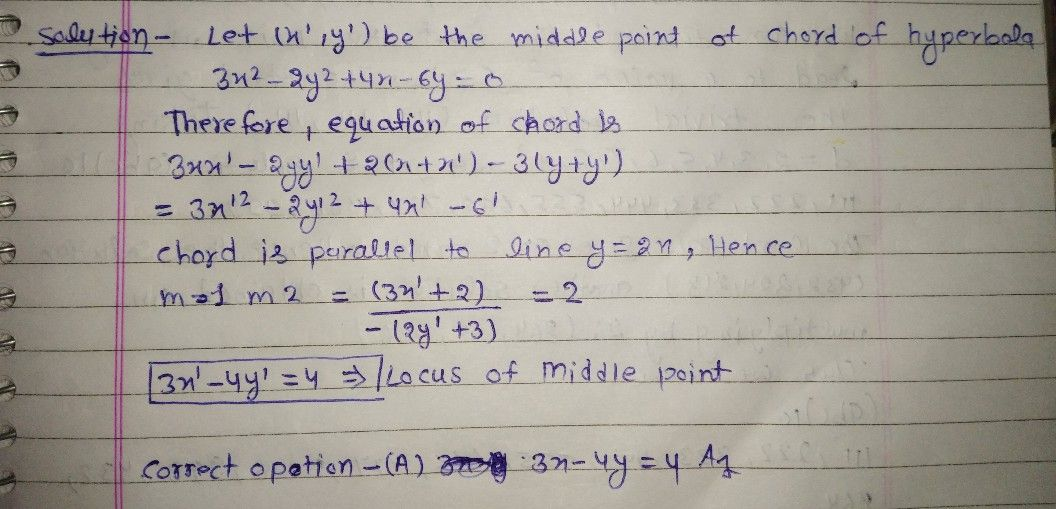Symbol
ProblemThe locus of the middle points of chords of hyperbola $3x^{2}-2y^{2}+4x-6y=0$ 0,parallel to $0y=2\times 1s$ $\left(A\right)$ $3\times -4y=4$ $\left($ (B) $8\right)$ $3y-4x+4=0$ $\left($ (C) $5\right)$ $4x-4y=3$ $\left($ (D) $\right)$ $3\times -4y=2$
10th-13th grade
Other
SolutionQanda teacher - vijay1997